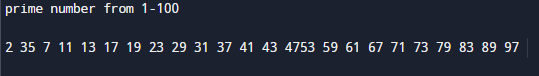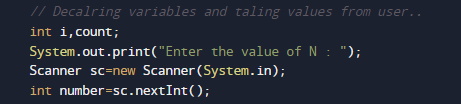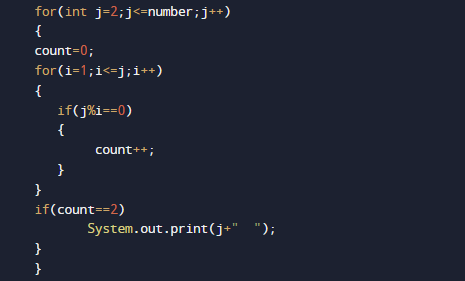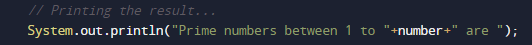# Java Program to display prime numbers between 1 and 100 or 1 and n

In this tutorial you will learn about the Java Program to display prime numbers between 1 and 100 or 1 and n and its application with practical example.

In this tutorial, we will learn to create a Java Program to display prime numbers between 1 and 100 or 1 and n using Java programming.

## Prerequisites

Before starting with this tutorial we assume that you are best aware of the following Java programming topics:

• Java Operators.
• Basic Input and Output function in Java.
• Class and Object in Java.
• Basic Java programming.
• If-else statements in Java.
• For loop in Java.
• User Define functions

## What is Prime Number?

A  number is called a Prime number if a number is divisible by 1 or itself only.
For example 2, 3, 5, 7, 11, 13, 17..are some prime numbers.

## Java Program to display prime numbers between 1 and 100 or 1 and n

In this program we would  print the prime numbers between 1 and 100 .First of all we take the  value of N form user and find prime number from 1 to N.Let have a look at the code.

## Output

Prime number From 1 – N.Prime number From 1 – 50In the above program, we have first declared and initialized a set variables required in the program.

• number= it will hold entered number.
• count=  it will show number is prime or not
• i and j = for iteration.

After declaring variables we take a value from user and print prime number from 1 – N.### Algorithm

• 1: START
• 2: declare variables count =0, number, i=1,j=1.
• 3: Take value of number from user
• 4: SET j= 1
• 5: SET count = 0
• 6: if i%j = = 0 then count ++
• 7: j = j + 1
• 8: if count= 2 then print the value of i.
• 9: number++.
• 10: i = i +1
• 11: ENDSimply here we check each and every value form 1 to N and print only values those satisfied the logic of a prime number it means number which divisible by itself only are prime numbers..and in that manner we print all prime number between 1 – n or 100.

In this tutorial we have learn about the Java Program to display prime numbers between 1 and 100 or 1 and n and its application with practical example. I hope you will like this tutorial.